# Double well levels

For the double well potential, the WKB approximation can be applied separately in each well and in the barrier region between the wells. The resulting energy levels depend on the width and depth of the wells, the height and width of the barrier, and the mass of the particle. In general, the energy levels are split into pairs of symmetric and antisymmetric states, with the symmetric states having lower energy than the antisymmetric states.

A particle in symmetrical wells, as shown in the figure, can move from one well to the other by tunneling past the barrier of its particular arrangement.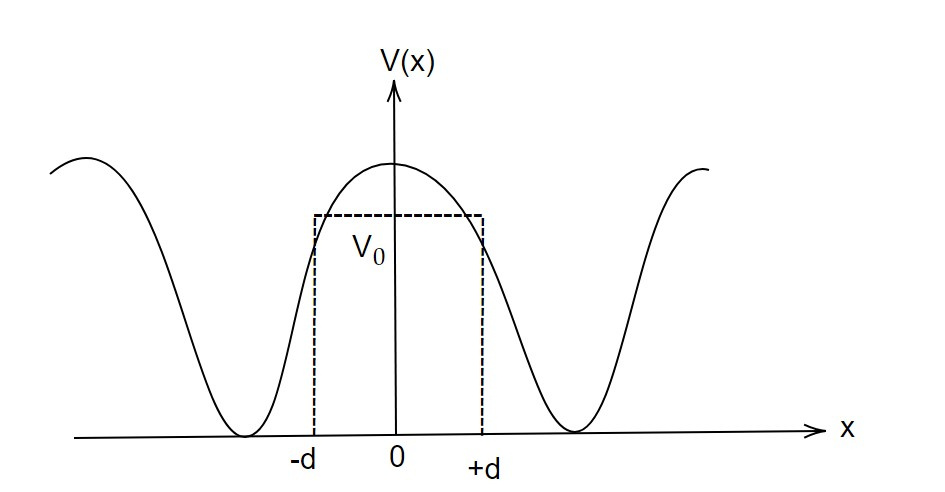Let the WKB approximation wavefunction is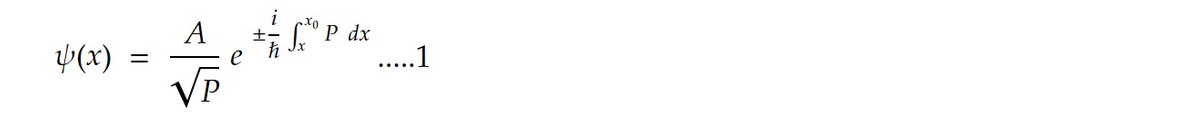which is the normalized WKB wavefunction for the particle of energy E. It will exponentially decay on either sides of the right and left wells.

The energy of the particles E will split into two levels Ee as Ψ (+x) and Ψ (-x). The linear combination of these wavefunction are also allowed wavefunction for the double walls.

Ψe(x) = 1/√2 [ Ψ (+x) + Ψ (-x)] .........2

And Ψo(x) = 1/√2 [ Ψ (+x) -Ψ (-x)] .........3

Being exponentially damped, the odd wave function Ψ (-x) is very small in the right well as compared to Ψ (+x) and vice versa for the left well.

But the product Ψ (+x)Ψ (-x) is negligible everywhere.

We have, the one dimension of Schrodinger equation as given by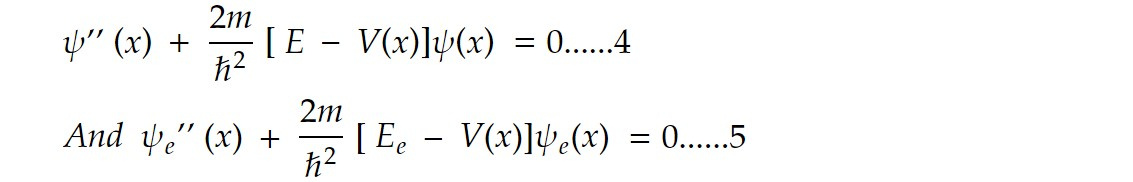Multiplying 4 by Ψe (x) and 5 by Ψ (x) and then subtracting we getIntegrating 6 over x between the limit 0 to ∞ we have,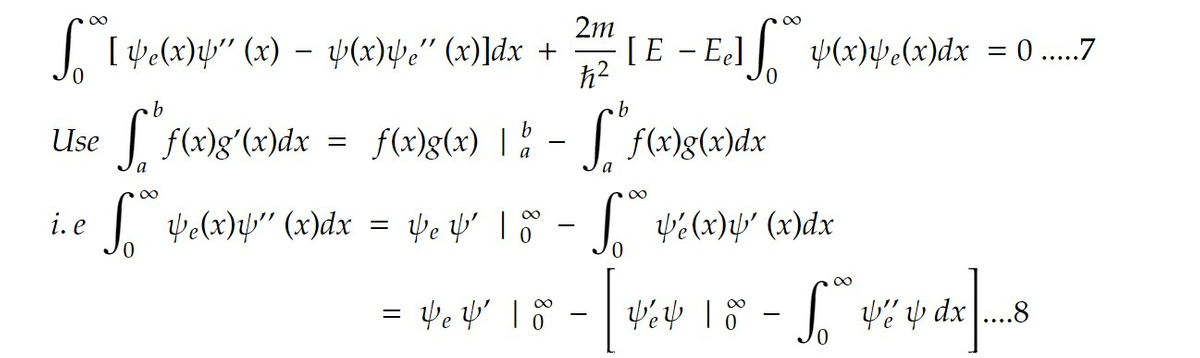Again setting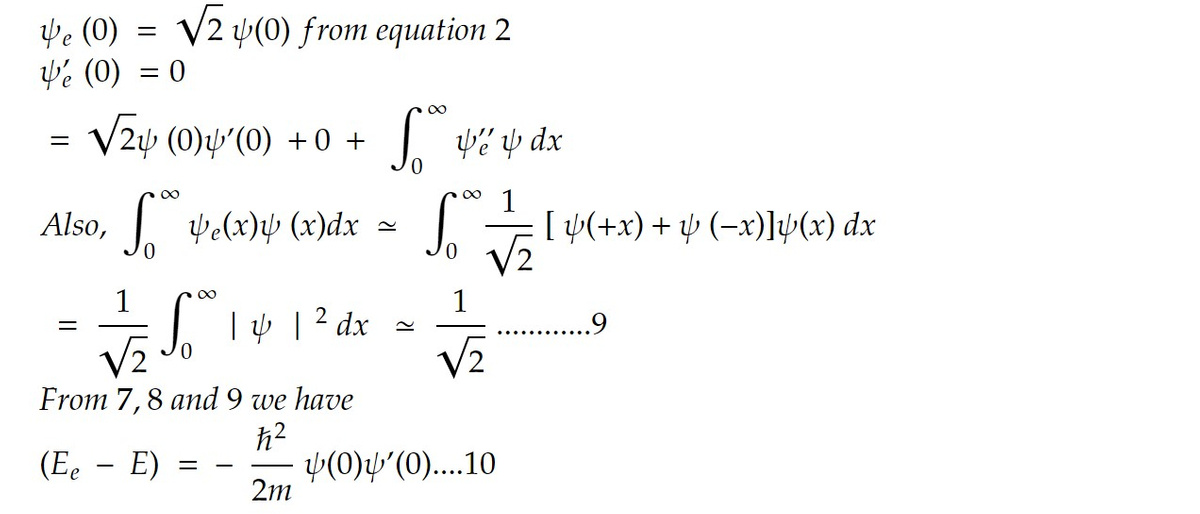Similarly for odd function i.e for antisymmetric case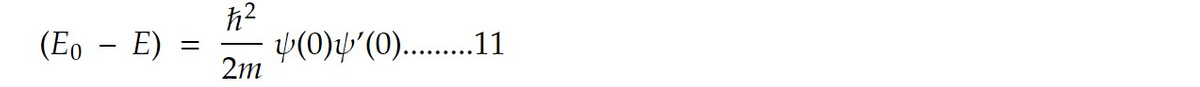Subtracting 10 from 11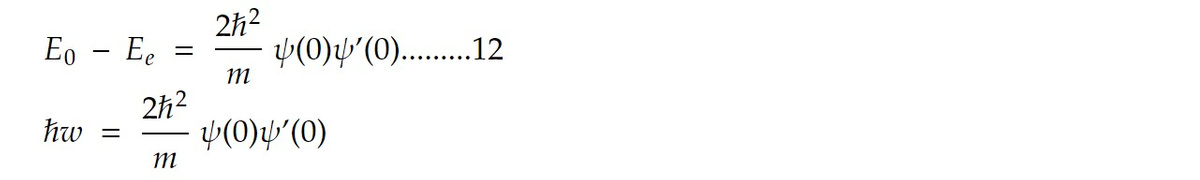where w is the inversion frequency

Also the WKB wavefunction for the non classical region we have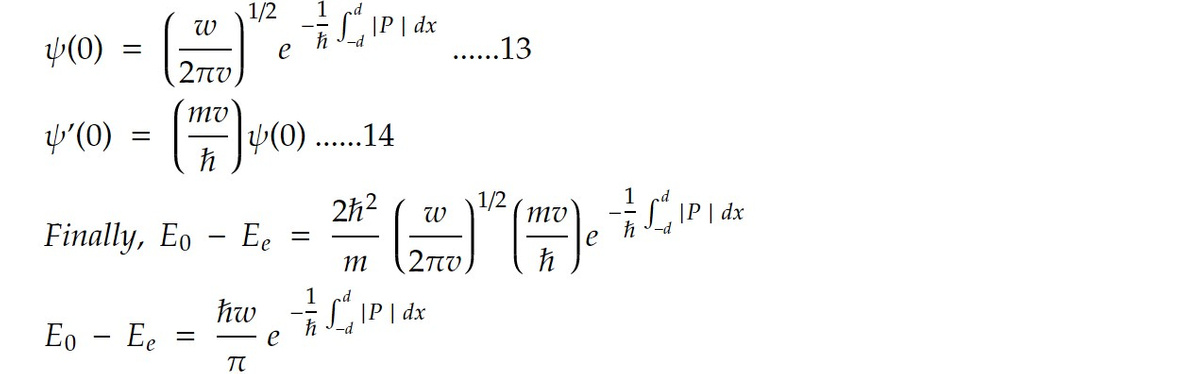The WKB approximation can be a useful tool for analyzing the energy levels and wave functions of particles in complex potentials, but it is an approximation and may not be accurate in all cases. Other methods, such as numerical solutions of the Schrödinger equation or perturbation theory, may be needed for more accurate results.

Previous note: WKB approximation for Barrier Tunneling

This note is a part of the Physics Repository.